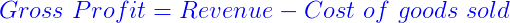# Gross Profit Formula

The gross profit formula is the total revenue minus cost of things sold. It is the company’s profit before all interest and tax payments. Gross profit is also called gross margin. Find below the formula to calculate the gross profit of a company.

## Formula for Gross Profit

The gross profit formula is given as:

 Gross Profit = Revenue – Cost of Goods Sold

Also Check: Profit Calculator### Solved Examples

Example 1: If the cost of a fan is 5.30 dollar and sold at 7.90 dollars. Find the gross profit?

Solution:

Given Revenue =

\(\begin{array}{l}5.30

Selling price =

\(\begin{array}{l}7.90

Gross Profit = Revenue – Cost of Goods

Gross Profit = 7.90 – 5.30

Gross Profit =

\(\begin{array}{l}2.6

Example 2: If the cost of a toy is 6.70 dollar and sold at 10.00 dollar. Find the gross profit?

Solution:

Given Revenue =

\(\begin{array}{l}6.70

Selling price =

\(\begin{array}{l}10

Gross Profit = 10 – 6.70

Gross Profit =

\(\begin{array}{l}3.3

Stay tuned with BYJU’S for more such interesting articles.